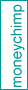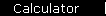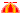### The Rule of 72 - Why it Works

The rule of 72 applies to annually compounded interest, but it's easiest to understand by looking at the case of continuously compounded interest first. We'll write P for the starting principal and r for the return rate (as a decimal); we're looking for Y to double P:

2P = PeYr

Solve for Y:

Y = ln(2) / r

The log of 2 is about equal to .69, so

Y = .69 / r

You can think of this as The Rule of 69 (multiplying the .69 by one hundred, so that the interest rate can be expressed as a percent instead of a decimal). It isn't an estimate - it's the exact answer for doubling your money, assuming that the interest is compounded continuously. It's valid for any value of r.Solving the formula for annually compounded interest is messier:

2P = P(1 + r)Y

Y = ln(2) / ln(1 + r)

We want to approximate this as a neat fraction again,

Y = K / r

where K is some number that will make the approximation pretty good for some ranges of r (and pretty lousy for others). We'll choose K to make the approximation work for a return rate of ten percent:

ln(2) / ln(1 + r) = K / r

ln(2) / ln(1 + .1) = K / 0.1

K = [ln(2) / ln(1.1)] x 0.1

K = .727

Now 72.7 is really closer to 73 than 72, so why isn't it The Rule of 73? Well... uh, the continuously compounded case gives you 69, so you want to round the 72.7 down instead of up... um, plus, 72 is easier to work with, since it's divisible by 12... Anyway, it's only an estimate. So, 72 it is!

Incidentally, if you repeat the above analysis for 8% instead of 10%, you do get a K that rounds to 72 instead of 73:

 Interest Rate: % Resulting Rule K x 100:

Back to the Rule of 72.

 home  |  features  |  glossary  |  calculator  |  about us  |  books